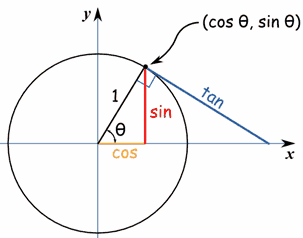## Monday, May 16, 2016

### Radar Robot! Displaying a robot's radar on a PC with Processing.

This is my super cool BOE BOT platform that I've converted from PBasic to Arduino! I used him to test out a lot of ideas I have. This weekend I slapped on a Ping Sonar to hi front Servo and turned him into a portable Sonar detector for my laptop.

Radar Robot scans the room with an ultrasonic sensor and then displays the layout of the room as a graphical radar on my computer. It works by rotating a Ping ultrasonic sensor on top of a servo 180 degrees.

The robot sends a command to the servo to rotate one degree forward. Then it takes a distance reading from the Ping ultrasonic sensor. The two values being used are angle and distance. We could save both these values in two different arrays. However, since the angle we are reading will be predictably from 0 to 180 degrees. we can save the distance readings in an array of size 181 (we're including 0th degree).

Index 90 of distanceArray will be the distance reading taken at angle 90 on the servo.

The distance is measured using the Ping sensor. We know the angle of the servo, because the Arduino is telling it what angle to face. With these two variables we have all the information we need to draw a radar.

First, lets see the raw data that the Arduino is storing.

distanceArray
Index 1  3cm
Index 2 : 4cm
...
Index90 :10cm
Index 91: 10cm
...
Index 180: 3cm

If we put it into excel, you can start to visualize where the object is. The shorter bars mean less distance, which means the object is closer. The problem with this layout though, is that it is linear. The far left is really pointing to 90 degrees left, the center is straight forward and the rightmost side is 90 degrees right.In this example, the robot is looking around the table with a laptop on one side a cup on the other.
The sides are where the farthest points are and where SonarBot can see the farthest ahead of him. It's difficult to visualize this in this format. We need to make this data into a circle like on a radar.

What do we want to do?
Input: Angle, Distance
Output: A line that rotates around a 180 degree half circle and gets longer with distance.

How do we get from distance and angle to a circle?

Easy! Right angle triangles!Look familiar? If you ever asked yourself when you ever need to use Trig in real life, this is the moment.

First we take the angle that our servo is facing, skipping 0 and starting at 1 degree, and apply both the sin() and cos() function to it. This will return the Cartesian coordinates on the circle in a value from 0 to 1.

For example, angle +45 will return Cartesian coordinates (0.70, 0.70).
Next, we multiply both the Cartesian coordinates by the distance of that the sonar has stored for that angle. That will scale it so that an object at 90 degrees and 10 inches in front of the robot will be a point in the center of the screen and 10 inches up. Next, all we have to do is a program a line from origin (0,0) to the point of object, and repeat for the next step.*

Now you've got a sonar robot!

*In reality, our point of origin should be (Screen X middle, Screen Y bottom). Also since our coordinates assume a center origin, we can just add the x distance to (ScreenWidth / 2) and it will subtract when it is negative.

Also, since our screen's Y value starts at the top, we should subtract the Y coordinate from Screen Height so it is at the bottom.